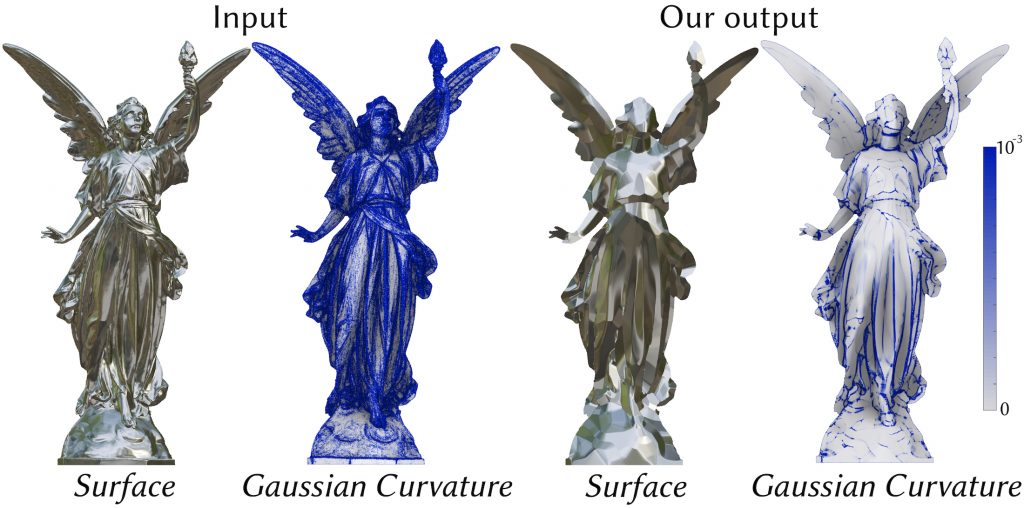# Developability of Heightfields via Rank MinimizationSilvia Sellán

Noam Aigerman

Alec Jacobson

SIGGRAPH 2020

A surface is called developable if it can be obtained by bending a planar surface without stretching it. Mathematically, this means it’s an isometry of the plane. A common alternative definition for a developable surface is one that has zero Gaussian curvature everywhere. We start by observing that a zero Gaussian curvature means a zero determinant of the surface’s Second Fundamental Form, which in the case of heightfield surfaces means a zero determinant of the heightfield’s Hessian. Since the Hessian is a 2 by 2 matrix, a zero determinant means its rank is either 1 or 0. This allows us to look at developability as a rank minimization problem, which we convexify using the tools of Rank Minimization and Compressed Sensing. We solve this convex problem using a tailor-made ADMM, and this lets us recover developable outputs from originally noisy (real!) scanned signals. We can also developablize surfaces that are far from being developable and we can even pose problems like finding the most developable surface satisfying certain constraints.